# Circle and Terms related to it.pptx

Mar. 29, 2023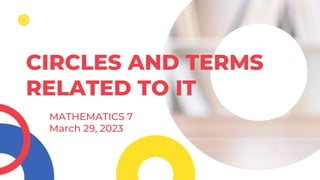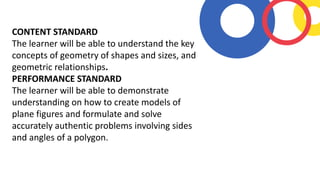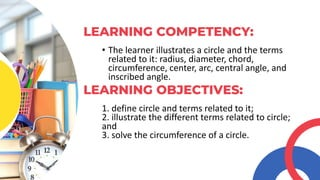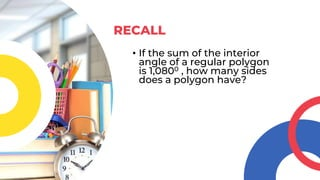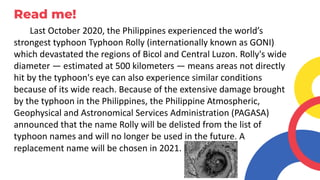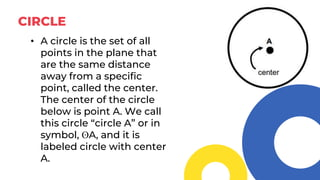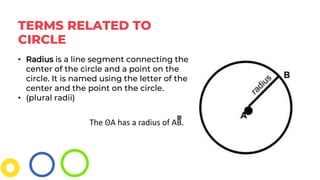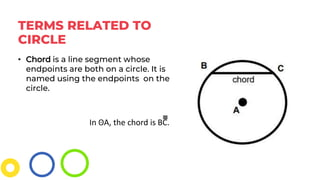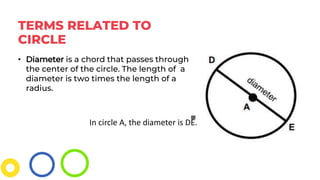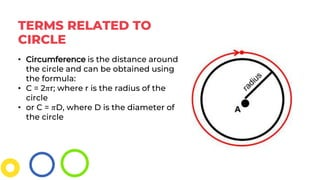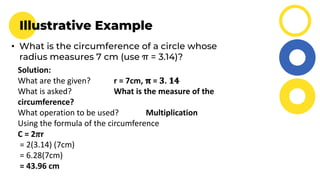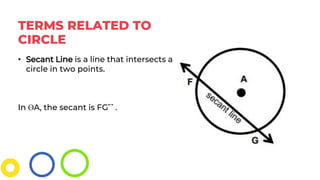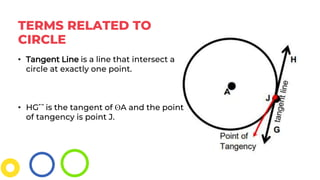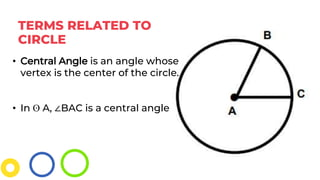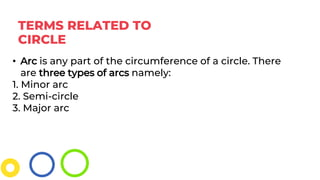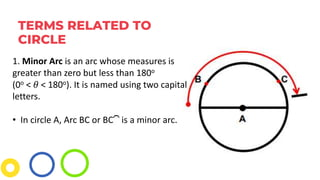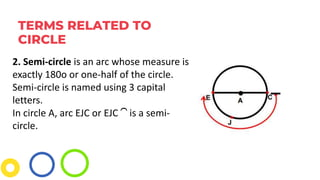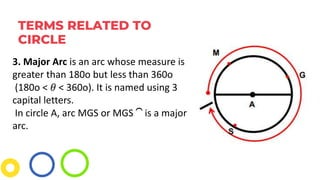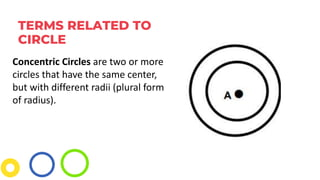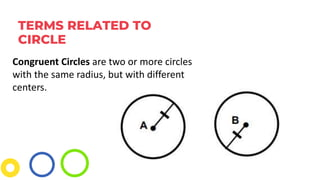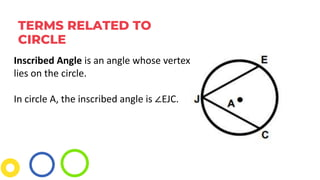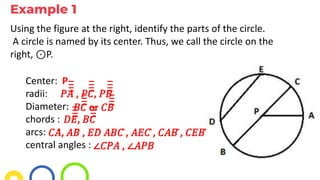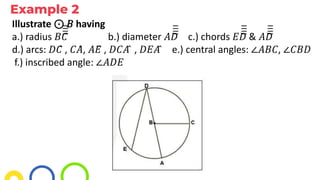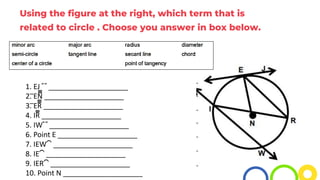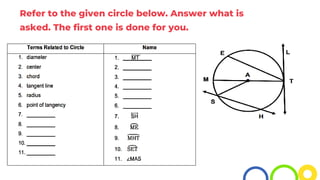1 of 26

### Circle and Terms related to it.pptx

1. CIRCLES AND TERMS RELATED TO IT MATHEMATICS 7 March 29, 2023
2. CONTENT STANDARD The learner will be able to understand the key concepts of geometry of shapes and sizes, and geometric relationships. PERFORMANCE STANDARD The learner will be able to demonstrate understanding on how to create models of plane figures and formulate and solve accurately authentic problems involving sides and angles of a polygon.
3. • The learner illustrates a circle and the terms related to it: radius, diameter, chord, circumference, center, arc, central angle, and inscribed angle. LEARNING COMPETENCY: 1. define circle and terms related to it; 2. illustrate the different terms related to circle; and 3. solve the circumference of a circle. LEARNING OBJECTIVES:
4. • If the sum of the interior angle of a regular polygon is 1,0800 , how many sides does a polygon have? RECALL
5. Read me! Last October 2020, the Philippines experienced the world’s strongest typhoon Typhoon Rolly (internationally known as GONI) which devastated the regions of Bicol and Central Luzon. Rolly's wide diameter — estimated at 500 kilometers — means areas not directly hit by the typhoon's eye can also experience similar conditions because of its wide reach. Because of the extensive damage brought by the typhoon in the Philippines, the Philippine Atmospheric, Geophysical and Astronomical Services Administration (PAGASA) announced that the name Rolly will be delisted from the list of typhoon names and will no longer be used in the future. A replacement name will be chosen in 2021.
6. CIRCLE • A circle is the set of all points in the plane that are the same distance away from a specific point, called the center. The center of the circle below is point A. We call this circle “circle A” or in symbol, ʘA, and it is labeled circle with center A.
7. TERMS RELATED TO CIRCLE • Radius is a line segment connecting the center of the circle and a point on the circle. It is named using the letter of the center and the point on the circle. • (plural radii) The ʘA has a radius of AB̅̅̅̅̅̅̅̅̅̅̅̅̅̅̅̅.
8. TERMS RELATED TO CIRCLE • Chord is a line segment whose endpoints are both on a circle. It is named using the endpoints on the circle. In ʘA, the chord is BC̅̅̅̅̅̅̅̅̅̅̅̅̅̅̅̅.
9. TERMS RELATED TO CIRCLE • Diameter is a chord that passes through the center of the circle. The length of a diameter is two times the length of a radius. In circle A, the diameter is DE̅̅̅̅̅̅̅̅̅̅̅̅̅̅̅̅.
10. TERMS RELATED TO CIRCLE • Circumference is the distance around the circle and can be obtained using the formula: • C = 2𝜋r; where r is the radius of the circle • or C = 𝜋D, where D is the diameter of the circle
11. Illustrative Example • What is the circumference of a circle whose radius measures 7 cm (use π = 3.14)? Solution: What are the given? r = 7cm, 𝛑 = 𝟑. 𝟏𝟒 What is asked? What is the measure of the circumference? What operation to be used? Multiplication Using the formula of the circumference C = 2𝜋r = 2(3.14) (7cm) = 6.28(7cm) = 43.96 cm
12. TERMS RELATED TO CIRCLE • Secant Line is a line that intersects a circle in two points. In ʘA, the secant is FG⃡ .
13. TERMS RELATED TO CIRCLE • Tangent Line is a line that intersect a circle at exactly one point. • HG⃡ is the tangent of ʘA and the point of tangency is point J.
14. TERMS RELATED TO CIRCLE • Central Angle is an angle whose vertex is the center of the circle. • In ʘ A, ∠BAC is a central angle
15. TERMS RELATED TO CIRCLE • Arc is any part of the circumference of a circle. There are three types of arcs namely: 1. Minor arc 2. Semi-circle 3. Major arc
16. TERMS RELATED TO CIRCLE 1. Minor Arc is an arc whose measures is greater than zero but less than 180o (0o < 𝜃 < 180o). It is named using two capital letters. • In circle A, Arc BC or BC⏜ is a minor arc.
17. TERMS RELATED TO CIRCLE 2. Semi-circle is an arc whose measure is exactly 180o or one-half of the circle. Semi-circle is named using 3 capital letters. In circle A, arc EJC or EJC ⏜ is a semi- circle.
18. TERMS RELATED TO CIRCLE 3. Major Arc is an arc whose measure is greater than 180o but less than 360o (180o < 𝜃 < 360o). It is named using 3 capital letters. In circle A, arc MGS or MGS ⏜ is a major arc.
19. TERMS RELATED TO CIRCLE Concentric Circles are two or more circles that have the same center, but with different radii (plural form of radius).
20. TERMS RELATED TO CIRCLE Congruent Circles are two or more circles with the same radius, but with different centers.
21. TERMS RELATED TO CIRCLE Inscribed Angle is an angle whose vertex lies on the circle. In circle A, the inscribed angle is ∠EJC.
22. Example 1 Using the figure at the right, identify the parts of the circle. A circle is named by its center. Thus, we call the circle on the right, ⊙P. Center: radii: Diameter: chords : arcs: central angles : P 𝑃𝐴̅̅̅̅̅̅̅̅̅̅̅̅̅̅̅̅ , 𝑃𝐶̅̅̅̅̅̅̅̅̅̅̅̅̅̅̅̅ , 𝑃𝐵̅̅̅̅̅̅̅̅̅̅̅̅̅̅̅̅ 𝐵𝐶̅̅̅̅̅̅̅̅̅̅̅̅̅̅̅̅ or 𝐶𝐵̅̅̅̅̅̅̅̅̅̅̅̅̅̅̅̅ 𝐷𝐸̅̅̅̅̅̅̅̅̅̅̅̅̅̅̅̅ , 𝐵𝐶̅̅̅̅̅̅̅̅̅̅̅̅̅̅̅̅ 𝐶𝐴̂, 𝐴𝐵̂ , 𝐸𝐷̂ 𝐴𝐵𝐶 ̂, 𝐴𝐸𝐶 ̂, 𝐶𝐴𝐵 ̂, 𝐶𝐸𝐵 ̂ ∠𝐶𝑃𝐴 , ∠𝐴𝑃𝐵
23. Example 2 Illustrate ⊙ 𝐵 having a.) radius 𝐵𝐶̅̅̅̅̅̅̅̅̅̅̅̅̅̅̅̅ b.) diameter 𝐴𝐷̅̅̅̅̅̅̅̅̅̅̅̅̅̅̅̅ c.) chords 𝐸𝐷̅̅̅̅̅̅̅̅̅̅̅̅̅̅̅̅ & 𝐴𝐷̅̅̅̅̅̅̅̅̅̅̅̅̅̅̅̅ d.) arcs: 𝐷𝐶̂ , 𝐶𝐴̂, 𝐴𝐸̂ , 𝐷𝐶𝐴 ̂ , 𝐷𝐸𝐴 ̂ e.) central angles: ∠𝐴𝐵𝐶, ∠𝐶𝐵𝐷 f.) inscribed angle: ∠𝐴𝐷𝐸
24. Using the figure at the right, which term that is related to circle . Choose you answer in box below. 1. EJ ⃡ ____________________ 2. ̅̅̅̅EN̅̅̅̅̅̅̅̅̅̅̅̅̅̅̅̅ ____________________ 3. ̅̅̅̅ER̅̅̅̅̅̅̅̅̅̅̅̅̅̅̅̅ ____________________ 4. IR̅̅̅̅̅̅̅̅̅̅̅̅ ____________________ 5. IW⃡ ____________________ 6. Point E ____________________ 7. IEW⏜ ____________________ 8. IE⏜ ____________________ 9. IER⏜ ____________________ 10. Point N ____________________
25. Refer to the given circle below. Answer what is asked. The first one is done for you.
26. Thank You

### Editor's Notes

1. Circles are present in real life, both in the natural world and in manmade creations.
2. Center: 𝑃 radii: 𝑃𝐴̅̅̅̅ , 𝑃𝐶̅̅̅̅, 𝑃𝐵̅̅̅̅ diameter: 𝐵𝐶̅̅̅̅ or 𝐶𝐵̅̅̅̅ chords : 𝐷𝐸̅̅̅̅, 𝐵𝐶̅̅̅̅ arcs: 𝐶𝐴̂, 𝐴𝐵̂ , 𝐸𝐷̂ 𝐴𝐵𝐶 ̂, 𝐴𝐸𝐶 ̂, 𝐶𝐴𝐵 ̂, 𝐶𝐸𝐵 ̂ central angles : ∠𝐶𝑃𝐴 , ∠𝐴𝑃𝐵
3. What Is It 1. tangent line 2. radius 3. chord 4. diameter 5. secant line 6. point of tangency 7. major arc 8. minor arc circle - 9. semi 10. center of a circle
4. What’s More MT 1. ̅̅̅̅̅ 2. Point A ET 3. ̅̅̅̅ LT 4. TL⃡ or ⃡ AS 5. ̅̅̅̅ AM , ̅̅̅̅̅ AT , or ̅̅̅̅ Point T 6. 7. secant line 8. minor arc circle - 9. semi 10. major arc 11. central angl